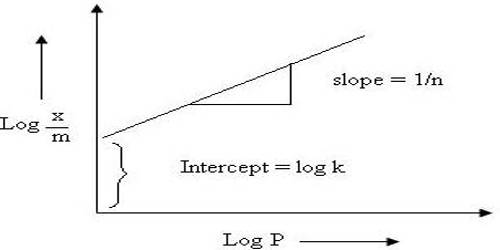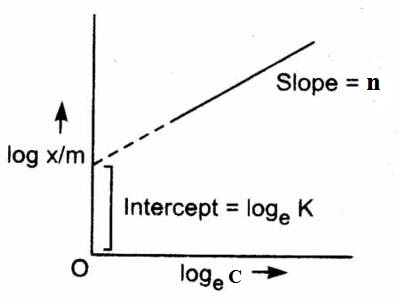Chemistry

# Freundlich IsothermFreundlich Isotherm

The Freundlich isotherm, presented by Freundlich in 1906 (Gaballah et al., 1997), was the first isotherm model proposed for sorption processes. It can be applied for nonideal sorption on heterogeneous surfaces, as well as, multilayer sorption. The extent of adsorption from solution is related to concentration by an empirical expression known as Freundlich isotherm.

x/m = KCn; where x is the amount of the solute adsorbed by m gram of the solid, C, the concentration and a and K are constants.

[The same isotherm can be used in gas/solid systems but it has been left out of the discussion for brevity and because it is a special form of Langmuir isotherm. No significant information can be obtained from it.]

This equation can be converted to a linear form by taking logarithms on both sides,

log (x/m) =log K + n log C

A plot of log (x/m) vs log C should give a straight line of slope n.An example is shown in Figure. Many systems show agreement with this equation over the limited range of concentrations, although the significance of n is not clearly understood; moreover, no useful or significant information can be obtained except establishing an empirical relation.

Limitation of Freundlich Isotherm

Experimentally it was determined that extent of adsorption varies directly with pressure till saturation pressure Ps is reached. Beyond that point rate of adsorption saturates even after applying higher pressure. Thus Freundlich Adsorption Isotherm failed at higher pressure.